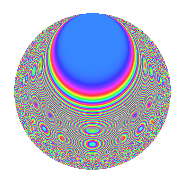# Properties

 Label 80.6.qLevel 80 Weight 6 Character orbit q Rep. character $$\chi_{80}(29,\cdot)$$ Character field $$\Q(\zeta_{4})$$ Dimension 116 Newform subspaces 1 Sturm bound 72 Trace bound 0

# Related objects

## Defining parameters

 Level: $$N$$ $$=$$ $$80 = 2^{4} \cdot 5$$ Weight: $$k$$ $$=$$ $$6$$ Character orbit: $$[\chi]$$ $$=$$ 80.q (of order $$4$$ and degree $$2$$) Character conductor: $$\operatorname{cond}(\chi)$$ $$=$$ $$80$$ Character field: $$\Q(i)$$ Newform subspaces: $$1$$ Sturm bound: $$72$$ Trace bound: $$0$$

## Dimensions

The following table gives the dimensions of various subspaces of $$M_{6}(80, [\chi])$$.

Total New Old
Modular forms 124 124 0
Cusp forms 116 116 0
Eisenstein series 8 8 0

## Trace form

 $$116q - 4q^{4} - 2q^{5} - 4q^{6} + O(q^{10})$$ $$116q - 4q^{4} - 2q^{5} - 4q^{6} + 532q^{10} - 4q^{11} + 1108q^{14} - 4q^{15} + 1216q^{16} + 2356q^{19} + 824q^{20} - 976q^{21} - 2864q^{24} - 12104q^{26} - 4q^{29} + 736q^{30} + 23056q^{31} - 38304q^{34} - 3864q^{35} - 20588q^{36} + 15144q^{40} - 25536q^{44} + 6734q^{45} - 36052q^{46} + 220884q^{49} + 56116q^{50} - 9472q^{51} - 104360q^{54} - 2552q^{56} + 14476q^{59} + 1008q^{60} - 48084q^{61} + 72944q^{64} - 27692q^{65} - 56736q^{66} - 23296q^{69} + 135260q^{70} - 186128q^{74} - 33244q^{75} - 35824q^{76} + 427312q^{79} - 129128q^{80} - 551132q^{81} - 418448q^{84} + 6248q^{85} + 177516q^{86} + 449576q^{90} - 231952q^{91} + 607356q^{94} + 38420q^{95} - 440264q^{96} + 406924q^{99} + O(q^{100})$$

## Decomposition of $$S_{6}^{\mathrm{new}}(80, [\chi])$$ into newform subspaces

Label Dim. $$A$$ Field CM Traces $q$-expansion
$$a_2$$ $$a_3$$ $$a_5$$ $$a_7$$
80.6.q.a $$116$$ $$12.831$$ None $$0$$ $$0$$ $$-2$$ $$0$$

## Hecke characteristic polynomials

There are no characteristic polynomials of Hecke operators in the database